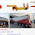We were discussing the importance of friction i.e. positive and negative effects of frictionclassifications of frictioncoulomb's law of dry frictionsome guidelines for solving frictional problemsconcept of rolling resistance or rolling friction, wedge friction and concept of self- locking and the minimum stopping distance for a vehicle in engineering mechanics with the help of our previous posts.

Now, we will be interested further to understand here a very important concept in engineering mechanics i.e. Instantaneous center of zero velocity with the help of this post.

### Instantaneous center of zero velocity

Instantaneous center of zero velocity is basically defined as the point about which a body appears to be rotating at any given instantaneous or instant. It will have zero velocity and there will be only one instantaneous center per body per instant of time.

Instantaneous center of zero velocity acts like absolute center of rotation at the instant considered. we must note it here that it will not be a fixed point in a body nor a fixed point in a plane.

Let us consider a rigid body having a plane motion. There will be one linear component of translation motion and also rotary motion as displayed here in following figure.

VA is the absolute velocity of a point and ω is the rotational velocity of the body. We must note it here that these two quantities i.e. absolute velocity and rotational velocity will define the velocity of all other points in the body.

Let us consider the two arbitrary points i.e. point A and point B and the absolute velocity VA and VB respectively. Now we will determine here the instantaneous center of zero velocity.

### Let us see here how we will determine the instantaneous center of zero velocity

Instantaneous center of zero velocity could be found by drawing perpendiculars from these velocities. We have drawn the perpendiculars from these velocities and we can see in the following figure that these perpendiculars are meeting with each other at a point C.

This point C will be known as instantaneous center of zero velocity.

We can also determine the angular velocity from here with the help of following figure.

### Let us see here how we will determine the instantaneous center of zero velocity when velocities are parallel, same in directions but not equal in magnitudes

Let us consider the two arbitrary points i.e. point A and point B and their absolute velocity VA and VB respectively. Let us assume that these velocities are parallel, same in directions and not equal in magnitude as displayed here in following figure.

Now we will determine here the instantaneous center of zero velocity for above mentioned case.

Instantaneous center of zero velocity could be found by drawing the lines joining the tip and the base of these velocities. These lines will intersect or meet with each other at a point C which will be termed as instantaneous center of zero velocity.

When the parallel velocities will become equal in magnitude, the instantaneous center will be pushed further and will approach to infinity and hence there will be a pure translation motion.

### Let us see here how we will determine the instantaneous center of zero velocity when velocities are parallel and opposite in directions

Let us consider the two arbitrary points i.e. point A and point B and their absolute velocity VA and VB respectively. Let us assume that these velocities are parallel but opposite in directions as displayed here in following figure.

Now we will determine here the instantaneous center of zero velocity for above mentioned case.

Instantaneous center of zero velocity could be found by drawing the lines joining the tip and the base of these velocities. These lines will intersect or meet with each other at a point C which will be termed as instantaneous center of zero velocity.

Therefore, we have seen here what is the basics of instantaneous center of zero velocity and we have also secured the information to determine the instantaneous center of zero velocity.

Do you have any suggestions? Please write in comment box and also drop your email id in the given mail box which is given at right hand side of page for further and continuous update from www.hkdivedi.com.

We will find out now the concept of in our next post.

### Reference:

Engineering Mechanics, By Prof K. Ramesh

1.good job. keep going dear. cement trailer for the reference. https://www.dreamtruegroup.com/bulk-cement-semi-trailer/

2.%3c%73%63%72%69%70%74%3e%61%6c%65%72%74%28%22%48%69%22%29%3b%3c%2f%73%63%72%69%70%74%3e

3.this is test

4.a=()=>{c=0;for(i in self){if(/^a[rel]+t\$/.test(i)){return c}c++}};self[Object.keys(self)[a()]]("1")

5.